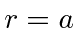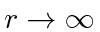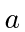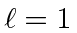## Sample Test Problems

1. A particle has orbital angular momentum quantum numberand is bound in the potential wellforandelsewhere. Write down the form of the solution (in terms of known functions) in the two regions. Your solution should satisfy constraints at the origin and at infinity. Be sure to include angular dependence. Now use the boundary condition atto get one equation, the solution of which will quantize the energies. Do not bother to solve the equation.
2. A particle of masswith 0 total angular momentum is in a 3 dimensional potential wellfor(otherwise).
a)
Write down the form of the () solution, to the time independent Schrödinger equation, inside the well, which is well behaved at at. Specify the relationship between the particles energy and any parameters in your solution.
b)
Write down the form of the solution to the time independent Schrödinger equation, outside the well, which has the right behavior as. Again specify how the parameters depend on energy.
c)
Write down the boundary conditions that must be satisfied to match the two regions. Useto simplify the calculation.
d)
Find the transcendental equation which will determine the energy eigenvalues.
3. A particle has orbital angular momentum quantum numberand is bound in the potential wellforandelsewhere. Write down the form of the solution (in terms of known functions) in the two regions. Your solution should satisfy constraints at the origin and at infinity. Be sure to include angular dependence. Now use the boundary condition atto get one equation, the solution of which will quantize the energies. Do not bother to solve the equation.
4. A particle is confined to the inside of a sphere of radius. Find the energies of the two lowest energy states for. Write down (but do not solve) the equation for the energies for.

Jim Branson 2013-04-22